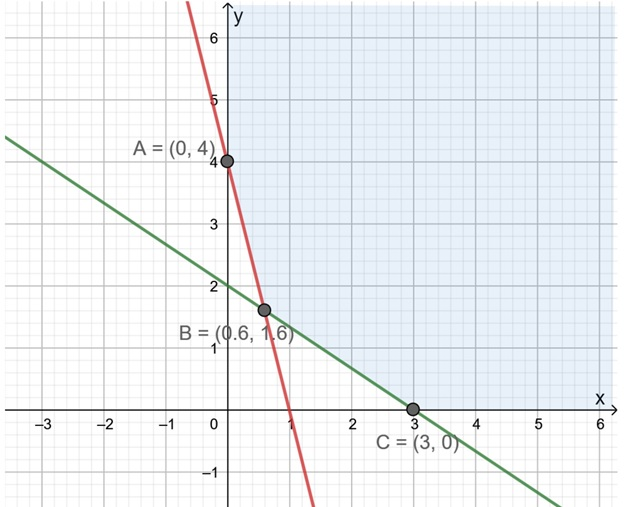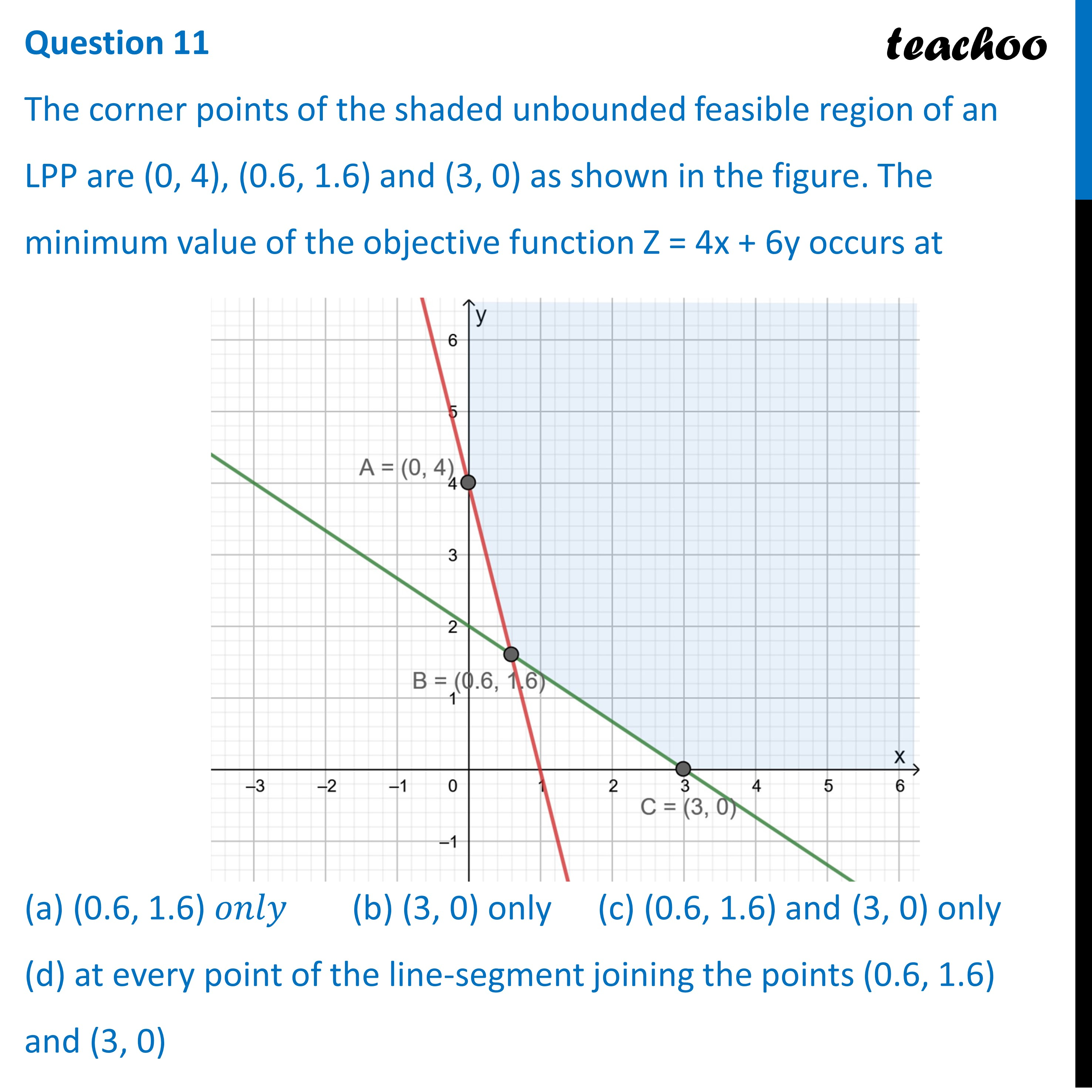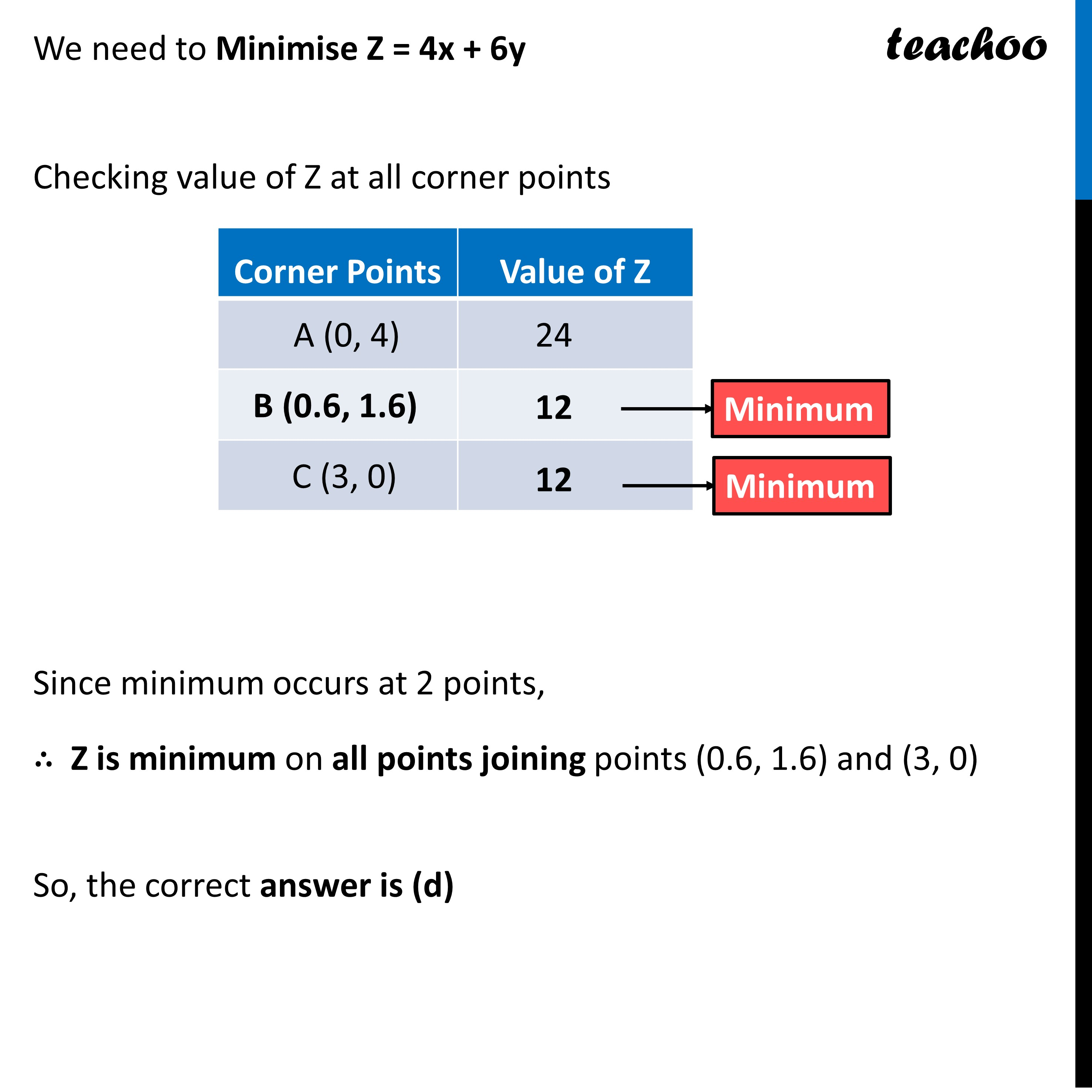CBSE Class 12 Sample Paper for 2023 Boards

Class 12
Solutions of Sample Papers and Past Year Papers - for Class 12 Boards

## The corner points of the shaded unbounded feasible region of an LPP are (0, 4), (0.6, 1.6) and (3, 0) as shown in the figure. The minimum value of the objective function Z = 4x + 6y occurs at## (d) at every point of the line-segment joining the points (0.6, 1.6) and (3, 0)

This question is Similar to Question-2 NCERT - Exemplar - MCQsGet live Maths 1-on-1 Classs - Class 6 to 12

### Transcript

Question 11 The corner points of the shaded unbounded feasible region of an LPP are (0, 4), (0.6, 1.6) and (3, 0) as shown in the figure. The minimum value of the objective function Z = 4x + 6y occurs at (a) (0.6, 1.6) 𝑜𝑛𝑙𝑦 (b) (3, 0) only (c) (0.6, 1.6) and (3, 0) only (d) at every point of the line-segment joining the points (0.6, 1.6) and (3, 0)We need to Minimise Z = 4x + 6y Checking value of Z at all corner points Since minimum occurs at 2 points, ∴ Z is minimum on all points joining points (0.6, 1.6) and (3, 0) So, the correct answer is (d)